# Analytical Reasoning (Test 4)

## Cat Entrance Exams : Data Interpretation And Logical Reasoning

| Home | | Cat Entrance Exams | | Data Interpretation And Logical Reasoning | | Analytical Reasoning |Analytical Reasoning
| Analytical Reasoning |
Q.1
Find the number of triangles in the given figure.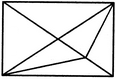A. 11
B. 13
C. 15
D. 17
Explaination / Solution:

The figure may be labelled as shown.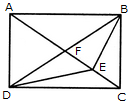The simplest triangles are AFB, FEB, EBC, DEC, DFE and AFD i.e. 6 in number.

The triangles composed of two components each are AEB, FBC, DFC, ADE, DBE and ABD i.e. 6 in number.

The triangles composed of three components each are ADC and ABC i.e. 2 in number.

There is only one triangle i.e. DBC which is composed of four components.

Thus, there are 6 + 6 + 2 + 1 = 15 triangles in the figure.

Workspace
Report
Q.2

Count the number of squares in the given figure.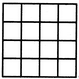A. 32
B. 30
C. 29
D. 28
Explaination / Solution:

The figure may be labelled as shown.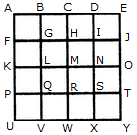The simplest squares are ABGF, BCHG, CDIH, DEJI, FGLK, GHML, HINM, IJON, KLQP, LMRQ, MNSR, NOTS, PQVU, QRWV, RSXW and STYX i.e. 16 in number.

The squares composed of four components each are ACMK, BDNL, CEOM, FHRP, GISQ, HJTR, KMWU, LNXV and MOYW i.e. 9 in number.

The squares composed of nine components each are ADSP, BETQ, FIXU and GJYV i.e. 4 in number.

There is one square AEYU composed of sixteen components.

There are 16 + 9 + 4 + 1 = 30 squares in the given figure.

Workspace
Report
Q.3

Find the number of quadrilaterals in the given figure.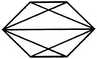A. 6
B. 7
C. 9
D. 11
Explaination / Solution:

The figure may be labelled as shown.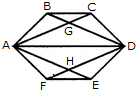The quadrilaterals in the figure are ABCD, ABDE, ABDF, ABDH, CDHA, CDEA, CDFA, DEAG, DEFA, FAGD and AGDH.

The number of quadrilaterals in the figure is 11.

Workspace
Report
Q.4

Count the number of squares in the given figure.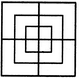A. 8
B. 12
C. 15
D. 18
Explaination / Solution:

The figure may be labelled as shown.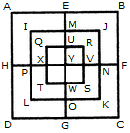The simplest squares are QUYX, URVY, YVSW and XYWT i.e. 4 in number.

The squares composed of two components each are IMYP, MJNY, YNKO and PYOL i.e. 4 in number.

The squares composed of three components each are AEYH, EBFY, YFCG and HYGD i.e. 4 in number.

There is only one square i.e. QRST composed of four components.

There is only one square i.e. IJKL composed of eight components.

There is only one square i.e. ABCD composed of twelve components.

Total number of squares in the given figure = 4 + 4 + 4+1 + 1 + 1 = 15.

Workspace
Report
Q.5

What is the minimum number of colours required to fill the spaces in the given diagram without any two adjacent spaces having the same colour?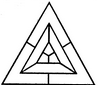A. 6
B. 5
C. 4
D. 3
Explaination / Solution:

The figure may be labelled as shown.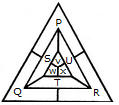The spaces P, Q and R have to be shaded by three different colours definitely (since each of these three spaces lies adjacent to the other two).

Now, in order that no two adjacent spaces be shaded by the same colour, the spaces T, U and S must be shaded with the colours of the spaces P, Q and R respectively.

Also the spaces X, V and W must be shaded with the colours of the spaces S, T and U respectively i.e. with the colours of the spaces R, P and Q respectively. Thus, minimum three colours are required.

Workspace
Report
Q.6

Count the number of triangles and squares in the given figure.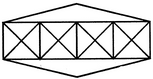A. 36 triangles, 7 squares
B. 38 triangles, 9 squares
C. 40 triangles, 7 squares
D. 42 triangles, 9 squares
Explaination / Solution:

The figure may be labelled as shown.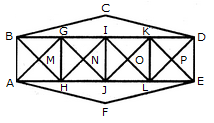Triangles:

The simplest triangles are BGM, GHM, HAM, ABM, GIN, UN, JHN, HGN, IKO, KLO, LJO, JIO, KDP, DEP, ELP, LKP, BCD and AFE i.e. 18 in number.

The triangles composed of two components each are ABG, BGH, GHA, HAB, HGI, GIJ, IJH, JHG, JIK, IKL, KLJ, LJI, LKD, KDE, DEL and ELK i.e. 16 in number.

The triangles composed of four components each are BHI, GJK, ILD, AGJ, HIL and JKE i.e. 6 in number.

Total number of triangles in the figure = 18 +16 + 6 = 40.

Squares :

The squares composed of two components each are MGNH, NIOJ and OKPL i.e. 3 in number.

The squares composed of four components each are BGHA, GIJH, IKLJ and KDEL i.e. 4 in number.

Total number of squares in the figure = 3 + 4 = 7.

Workspace
Report
Q.7

Count the number of triangles and squares in the given figure.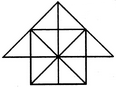A. 26 triangles, 5 squares
B. 28 triangles, 5 squares
C. 26 triangles, 6 squares
D. 28 triangles, 6 squares
Explaination / Solution:

The figure may be labelled as shown.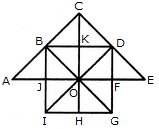Triangles:

The simplest triangles are JBO, BKO, KDO, DFO, FGO, GHO, HIO, IJO, ABJ, BCK, CKD and DEF i.e.12 in number.

The triangles composed of two components each are IBO, BDO, DGO, GIO, ABO, CDO, CBO, CBD and DEO i.e. 9 in number.

The triangles composed of four components each are IBD, BDG, DGI, GIB, ACO and COE i.e. 6 in number.

There is only one. triangle i.e. ACE composed of eight components.

Thus, there are 12 + 9 + 6 + 1 = 28 triangles in the given figure.

Squares:

The squares composed of two components each are BKOJ, KDFO, OFGH and JOHI i.e. 4 in number.

There is only one square i.e. CDOB composed of four components.

There is only one square i.e. BDGI composed of eight components.

Thus, there are 4 + 1 + 1 = 6 squares in the given figure.

Workspace
Report
Q.8

What is the minimum number of different colours required to paint he given figure such that no two adjacent regions have the same colour?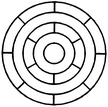A. 3
B. 4
C. 5
D. 6
Explaination / Solution:

The figure may be labelled as shown.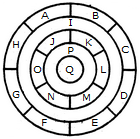The regions A, C, E and G can have the same colour say colour 1.

The regions B, D, F and H can have the same colour (but different from colour 1) say colour 2.

The region 1 lies adjacent to each one of the regions A, B, C, D, E, F, G and H and therefore it should have a different colour say colour 3.

The regions J, L and N can have the same colour (different from colour 3) say colour 1.

The regions K, M and O can have the same colour (different fromthe colours 1 and 3). Thus, these regions will have colour 2.

The region P cannot have any of the colours 1 and 2 as it lies adjacent to each one of the regions J, K, L, M, N and O and so it will have colour 3.

The region Q can have any of the colours 1 or 2.

Minimum number of colours required is 3.

Workspace
Report
Q.9

Count the number of triangles and squares in the given figure.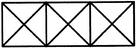A. 28 triangles, 3 squares
B. 24 triangles, 5 squares
C. 28 triangles, 5 squares
D. 24 triangles, 3 squares
Explaination / Solution:

The figure may be labelled as shown.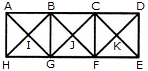Triangles :

The, simplest triangles are ABI, BGI, GHI, HAI, BCJ, CFJ, FGJ, GBJ, CDK, DEK, EFK and FCK i.e. 12 in number.

The triangles composed of two components each are ABG, BGH, GHA, HAB, BCF, CFG, FGB, GBC, CDE, DEF, EFC and FGD i.e. 12 in number.

The triangles composed of four components each are AGC, BFD, HBF and GCE i.e.4 in number.

Thus, there are 12 + 12 + 4 = 28 triangles in the given figure.

Squares :

The squares composed of two components each are BJGI and CKFJ i.e. 2 in number.

The squares composed of four components each are ABGH, BCFG and CDEF i.e. 3 in number.

Total number of squares in the figure = 2 + 3 = 5.

Workspace
Report
Q.10

Count the number of parallelogram in the given figure.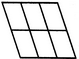A. 20
B. 18
C. 16
D. 12
Explaination / Solution:

The figure may be labelled as shown.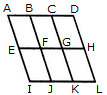The simplest ||gms are ABFE, BCGF, CDHG, EFJI, FGKJ and GHLK. These are 6 in number.

The parallelograms composed of two components each are ACGE, BDHF, EGKI, FHLJ, ABJI, BCKJ and CDLK. Thus, there are 7 such parallelograms.

The parallelograms composed of three components each are ADHE and EHLI i.e. 2 in number.

The parallelograms composed of four components each are ACKI and BDLJ i.e. 2 in number

There is only one parallelogram composed of six components, namely ADLI.

Thus, there are 6 + 7 + 2 + 2 + 1 = 18 parallelograms in the figure.

Workspace
Report